Home | | Pharmaceutical Drug Analysis | Complexometric Analysis: Theory

# Complexometric Analysis: Theory

1. Effect of pH on Complexation 2. Stability of Complexes 3. Colouration of Complexes 4. Titrability of Polyvalent Metal Ions Employing Disodium Edetate 5. Usage of pM Indicators in Complexometric Titrations

THEORY

Complex is a compound that is formed by the combination of a metal ion with a molecule that is capable of donating electrons, for example :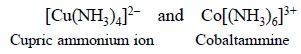In the above two examples both Cu2+ and Co2+ form complexes with lone pair of electrons present in the neutral molecule ammonia e.g., N’’H3.

Chelate is a complex that is formed by the combination of a polyvalent metal ion with a molecule which essentially contains two or more groups that can donate electrons.

Specifically, disodium ethylenediaminetetraacetate (EDTA) reacts with polyvalent metal ions to result in the formation of a fairly stable water-soluble complex, or a chelate compound.

It is, however, pertinent to mention here that the predominant state of the dissociated forms of EDTA (viz . Y4–, HY3– , H2Y2– and H3Y) is solely dependent upon the pH of the medium at which complexation takes place :

where, H4Y = ethylenediaminetetraacetic acid, and

Y4– = tetracetate ion.

In general, all EDTA complexation reactions essentially have the ratio of EDTA to metal ion as 1 : 1 Thus, we have :Ligand is a molecule that affords groups for attachment to metal ions such as EDTA.

Some examples of polyvalent metal ions are given below :

Bivalent Metal ions : Ca2+, Mg2+, Zn2+,

Trivalent Metal ions : Fe3+, Al3+, Cr3+,

Tetravalent Metal ions : Sn4+, Ce4+, Cr4+, Pt4+.

The structures of the complexes formed with di-, tri- and tetra-valent metal ions give rise to three, four and five rings respectively as depicted below :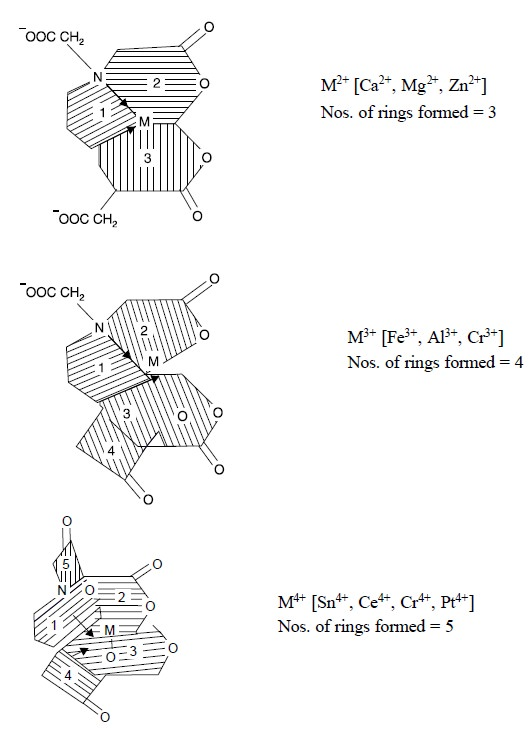There are various aspects in complex formation and detection, namely :

(i) Effect of pH on complexation,

(ii) Stability of complexes,

(iii) Colouration of complexes,

(iv) Titrability of polyvalent metal ions employing disodium edetate, and

(v) Usage of pM indicators in complexometric titrations.

## 1. EFFECT OF pH ON COMPLEXATION

Ethylenediamine tetracetic acid (H4Y) undergoes ionization at four, different stages, namely :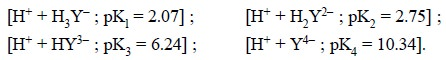In reality, the actual complexing species is the tetracetate ion i.e., Y4– ; therefore, complexation will take effect more efficiently and be more stable in an aikaline medium. Hence, it is evident that EDTA com-plexes of many divalent metals are quite stable in ammoniacal solution.

As we have seen earlier that the trivalent metal complexes are normally bound still more firmly due to the formation of four rings (unlike three rings with divalent metal complexes) and stable in strongly acidic solutions, for instance : cobalt (Co2+) EDTA complex is fairly stable in concentrated hydrochloric acid ( ~ 11.5 N).

Though a good number of metal-EDTA complexes are found to be quite stable over a wide-spectrum of pH, yet in actual practice solutions are normally buffered for two specific reasons :

(a) to stabilize the complex formed, and

(b) to achieve the most distinct colour-change of the indicator.

## 2. STABILITY OF COMPLEXES

Generally, the formation of a 1 : 1 chelate complex (MX) may be designated by the following equation :where, M = Metal ion, and

X = Chelating ion.

Hence, the stability constant, K, may be expressed as :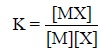where, items within the ‘square brackets’ represent activities.

There are two cardinal factors which influence the stability constant (K), namely :

(a) Elevation in temperature affords a slight enhancement in the ionization of the complex and a slight lowering of K, and

(b) Stability constant is decreased on the addition of electrolytes with no common ion ; whereas, ethyl alcohol enhances K, perhaps on account of the suppression of ionization.

Table 9.1, provides the values of the logarithms of stability constants (K) of EDTA-complexes of certain metals normally occurring in pharmaceutical substances :## 3. COLOURATION OF COMPLEXES

The formation of EDTA-metal ion complexes invariably attribute a change in the absorption spectrum pattern which ultimately forms the basis of a large number of colorimetric assays.

## 4. TITRABILITY OF POLYVALENT METAL IONS EMPLOYING DISODIUM EDETATE

Ethylenediamine tetracetic acid is found to be sparingly soluble in water ( ~ 0.2% w/v) whereas its corresponding disodium salt is almost 50 times more soluble than the parent compound (solubility ~ 10% w/v). Therefore, it is the disodium salt of EDTA which is normally used in complexometric titrations.

In actual practice, whenever the disodium EDTA solution is added to a solution of a metal ion previously buffered to augment complexation, it has been observed that initially the rate of change of concentration of metal ion is rather slow, but interestingly it picks up quite rapidly as further addition of sodium-EDTA approaches one equivalent.

## 5. USAGE OF pM INDICATORS IN COMPLEXOMETRIC TITRATIONS

The equivalence point in complexometric titrations is invariably observed by the help of pM indicators. The relationship amongst pM, concentrations of ligand, chelate complex and stability constant may be established by the following equations :

Assuming K as the stability constant, we have :Now, considering Eq. (a), if a solution is made in such a manner that [X] = [MX], we have :

pM = – pK

or pM = pK ... (b)

where, K is the dissociation constant.

From Eq. (b) it may be concluded that a solution having equal activities of free chelating agent and the metal-complex formed, the concentration of metal ions shall remain almost constant and would be buffered exactly in a similar fashion as are H+ ions in a pH-buffer. As we know that the various chelating agents are mostly basic in character, therefore, the equilibrium attained in a metal-buffer solution is largely influenced by a change in pH. Hence, it may be concluded that the amino acid type chelating agents, such as : ethylenediamine tetracetic acid and ammoniatriacetic acid, when [X] = [MX], pM increases proportionately with pH until it reaches a value pH 10, thereby attaining a constant value. Hence, this particular pH is the ‘Ideal pH’ at which complexometric titrations of metals with chelating agents in buffered solution must be performed.

pM Indicator : It is a dye that serves as a chelating agent to yield a dye-metal complex, which apparently differs in colour from the original dye, besides possessing a lower stability constant than the corresponding chelate-metal complex. Hence, the colour imparted to the solution is mostly attributed due to the dye-complex formed until the end-point, when an equivalent amount of sodium-EDTA has been incorporated. The critical point at which the metal-dye complex decomposes to yield free-dye on addition of the slightest excess of sodium-EDTA, is distinctly shown by a visible change in colour.

Examples :

(i)          Mordant Black 2 : (Syn. : Eriochrome Black T ; Solochrome Black T)(ii)       Murexide : (Syn. : Ammonium Purpurate)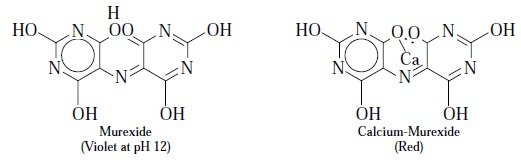Study Material, Lecturing Notes, Assignment, Reference, Wiki description explanation, brief detail
Pharmaceutical Drug Analysis: Complexometric Analysis : Complexometric Analysis: Theory |

Related Topics